• 一、Bar 条形图、 1、bar 函数、 2、矩阵数据表示、 3、bar 函数代码示例、 二、Bar 条形图样式、 1、bar 函数样式、 2、堆叠条形图示例、 三、水平条形图、 1、barh 函数、 2、代码示例、
文章目录一、Bar 条形图1、bar 函数2、矩阵数据表示3、bar 函数代码示例二、Bar 条形图样式1、bar 函数样式2、堆叠条形图示例三、水平条形图1、barh 函数2、代码示例

一、Bar 条形图

1、bar 函数

bar 函数参考文档 : https://ww2.mathworks.cn/help/matlab/ref/bar.html

2、矩阵数据表示

$x$ 值是一个矩阵 :
$x = \begin{bmatrix} 1 & 2 & 5 & 4 & 8 \end{bmatrix}$
代码表示例 :
% 条形图的数值列表
x = [1 , 2 , 5 , 4 , 8]


$y$ 是 $x$ 与另一个矩阵组成的新矩阵 :
$y = \begin{bmatrix} 1 & 2 & 5 & 4 & 8 \\\\ 1 & 2 & 3 & 4 & 5 \end{bmatrix}$
代码示例 :
% 数值列表 , 组成一个矩阵
y = [x; 1:5];


3、bar 函数代码示例

bar 函数代码示例 :
% 条形图的数值列表
x = [1, 2, 5, 4, 8];

% 数值列表 , 组成一个矩阵
y = [x; 1:5];

% 绘制第一张图像
subplot(3, 1, 1);

% 绘制条形图 , x 中每个元素对应一个条形
bar(x);

% 绘制第二张图像
subplot(3, 1, 2);

% 绘制条形图 , y 中每个元素对应一个条形
% y 是个矩阵 , 有 2 x 5 = 10 个元素
bar(y);

% 绘制第三张图像
subplot(3, 1, 3);

% 绘制立体 3D 条形图 , y 中每个元素对应一个条形
% y 是个矩阵 , 有 2 x 5 = 10 个元素
bar3(y);


代码示例 :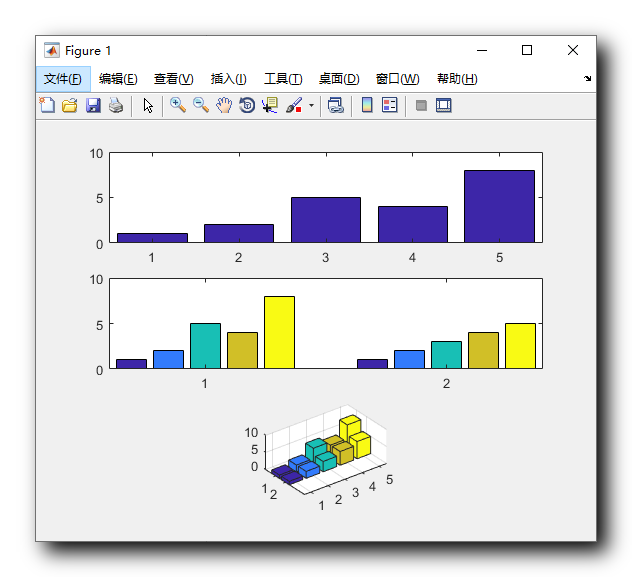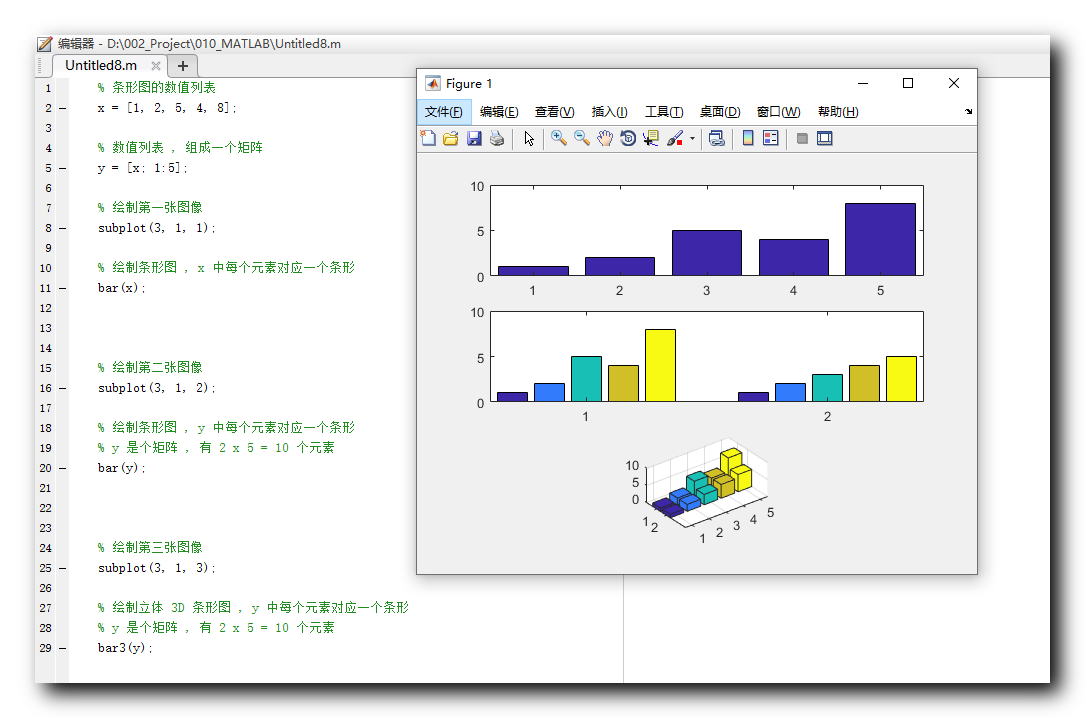二、Bar 条形图样式

bar 函数参考文档 : https://ww2.mathworks.cn/help/matlab/ref/bar.html

1、bar 函数样式

在 bar 函数的数据后面 , 可以使用字符串指定一个条形图样式 ,
条形图的四种样式如下 :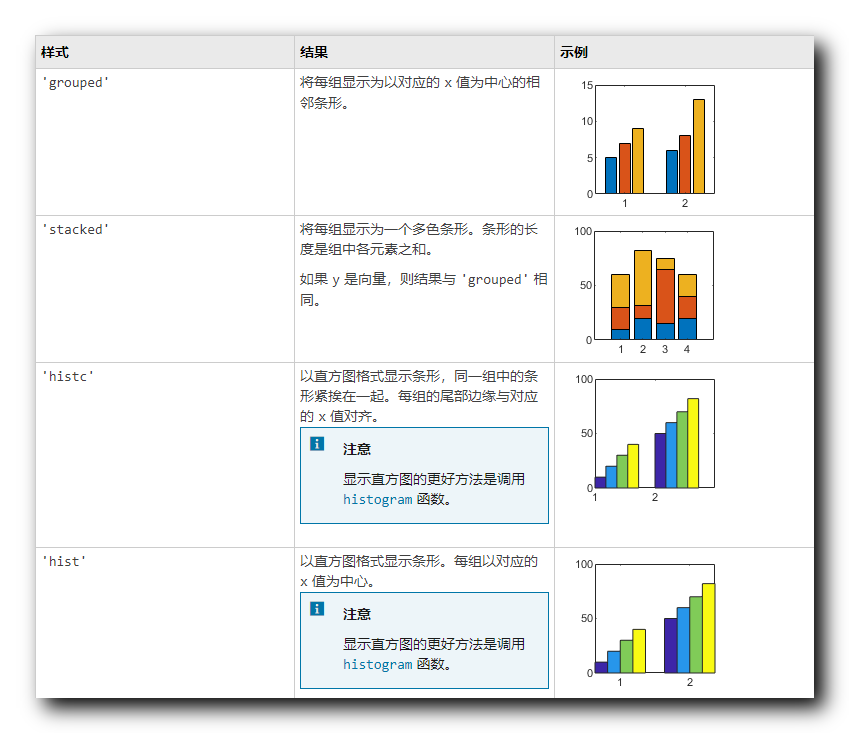2、堆叠条形图示例

% 条形图的数值列表
x = [1, 2, 5, 4, 8];

% 数值列表 , 组成一个矩阵
y = [x; 1:5];

% 绘制第一张图像
subplot(2, 1, 1);

% 绘制条形图 , y 中每个元素对应一个条形
% y 是个矩阵 , 有 2 x 5 = 10 个元素
bar(y);

% 绘制第二张图像
subplot(2, 1, 2);

% 绘制条形图 , y 中每个元素对应一个条形
% y 是个矩阵 , 有 2 x 5 = 10 个元素
bar(y, 'stacked');


绘图效果 :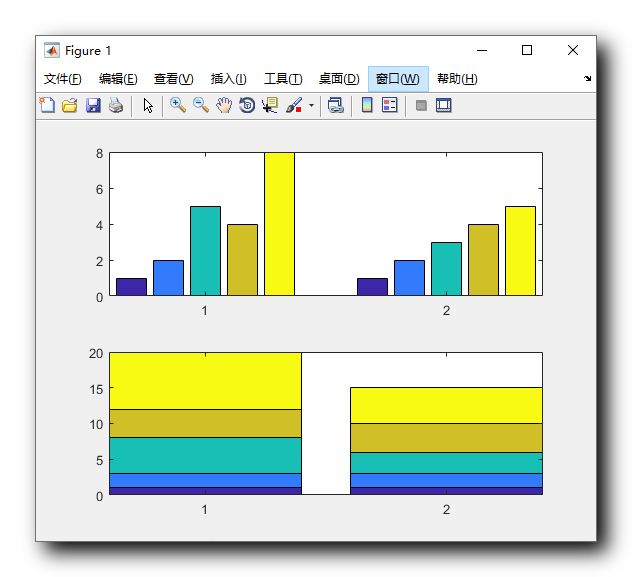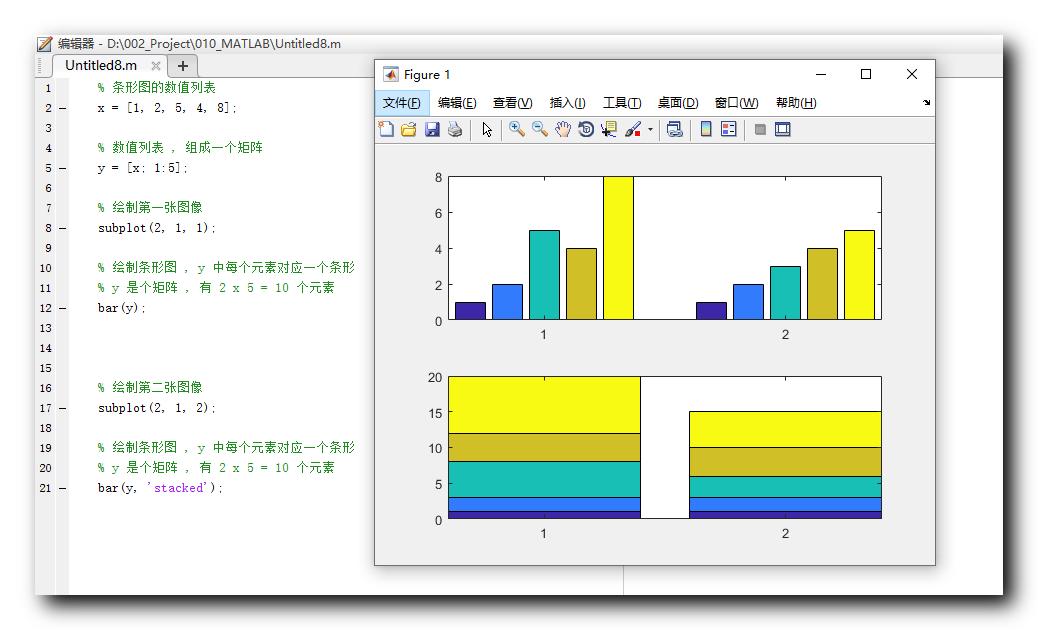三、水平条形图

barh 函数参考文档 : https://ww2.mathworks.cn/help/matlab/ref/barh.html

1、barh 函数

与 bar 用法类似 , 使用 barh 函数绘制的条形图是水平条形图 ;

2、代码示例

代码示例  :
% 条形图的数值列表
x = [1, 2, 5, 4, 8];

% 数值列表 , 组成一个矩阵
y = [x; 1:5];

% 绘制第一张图像
subplot(2, 1, 1);

% 绘制条形图 , y 中每个元素对应一个条形
% y 是个矩阵 , 有 2 x 5 = 10 个元素
barh(y);

% 绘制第二张图像
subplot(2, 1, 2);

% 绘制条形图 , y 中每个元素对应一个条形
% y 是个矩阵 , 有 2 x 5 = 10 个元素
barh(y, 'stacked');


绘图结果 :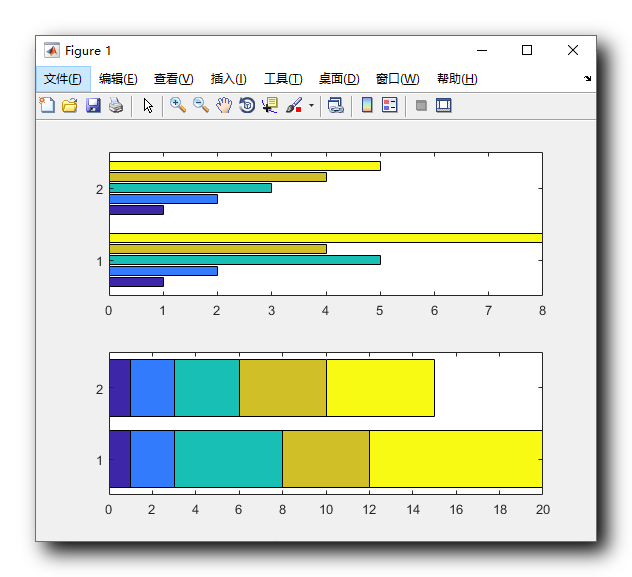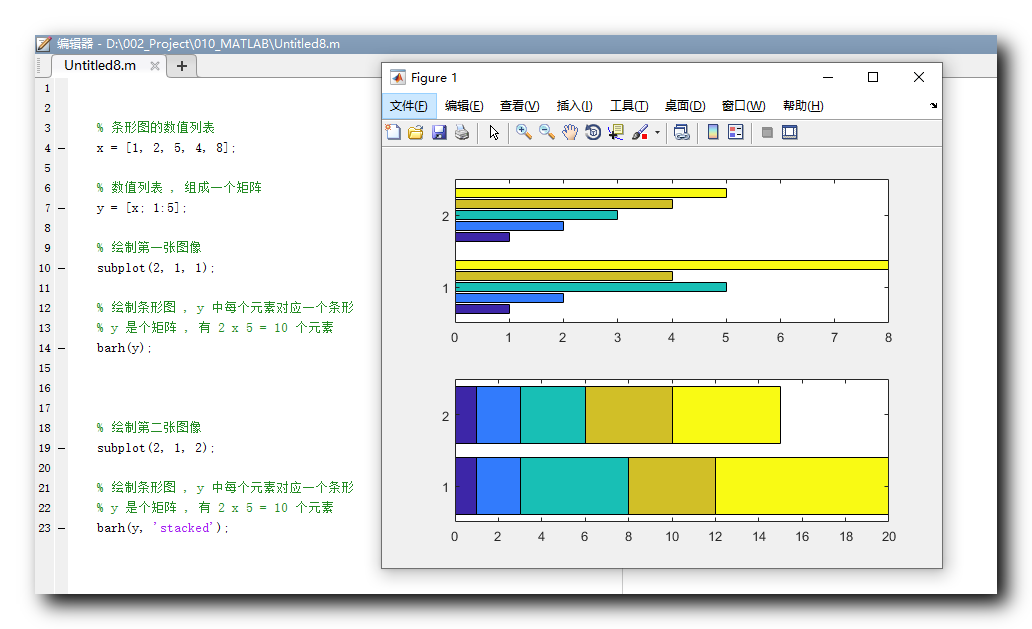展开全文• 一、Boxplot 箱线图、 1、boxplot 函数、 2、代码示例、 二、Error Bar 误差条线图、 1、errorbar 函数、 2、代码示例、
文章目录一、Boxplot 箱线图1、boxplot 函数2、代码示例二、Error Bar 误差条线图1、errorbar 函数2、代码示例

一、Boxplot 箱线图

1、boxplot 函数

boxplot 函数文档 : https://ww2.mathworks.cn/help/stats/boxplot.html

stairs 函数语法 :
boxplot(x)
boxplot(x,g)

boxplot(x) : 根据 x 中的数据创建箱线图 ;

x 是向量 : 绘制一个箱子 ;
x 是矩阵 : 为每个矩阵列绘制一个箱子 ;

箱子 :

中位数 : 每个箱子都有一个中心标记 , 表示中位数 ;
第 25 百分位数 : 箱子底边 ;
第 75 百分位数 : 箱子顶边 ;

boxplot(x,g) : g 中包含若干分组变量 ;

2、代码示例

代码示例 :
% 加载数据
% 不同国家中每加仑汽油能跑多少英里
load carsmall

% MPG 是箱线图数据
% Origin 中包含多个分组变量
boxplot(MPG, Origin);


绘图结果 :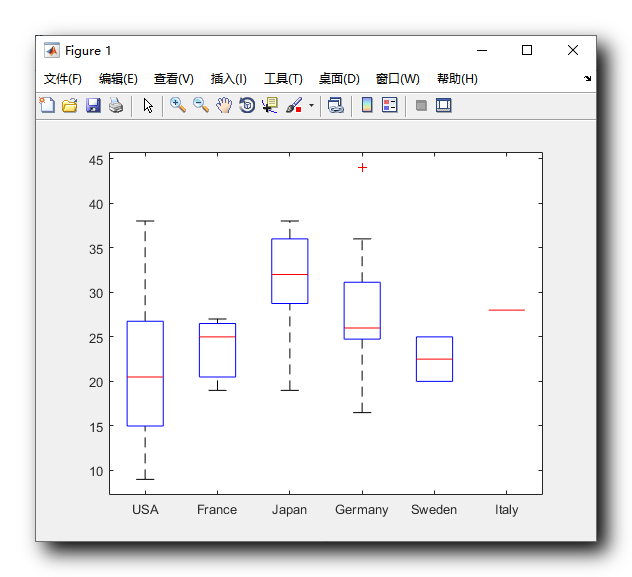二、Error Bar 误差条线图

1、errorbar 函数

errorbar 函数文档 :  https://ww2.mathworks.cn/help/matlab/ref/errorbar.html

errorbar 函数语法 :
errorbar(y,err)
errorbar(x,y,err)

errorbar(y,err) 与 plot(y) 绘制的曲线相同 , err 参数指的是对应点的误差范围大小 ;
errorbar(x, y,err) 与 plot(x, y) 绘制的曲线相同 , err 参数指的是对应点的误差范围大小 ;

整个竖条 , 是有可能出现的误差数值 , errorbar 的第三个参数就是该误差数值的区间大小 ;

2、代码示例

代码示例 :
% 生成 x 向量, 0 ~ pi , 步长 pi / 10
x = 0 : pi / 10 : pi;

% 生成 y 轴的值对应向量
y = sin(x);

% 生成 e 向量 , 表示每个对应 x 位置的误差范围
e = std(y) * ones(size(x));

% 绘制含误差条的线图
% e 表示误差范围
errorbar(x, y, e);


绘图效果 :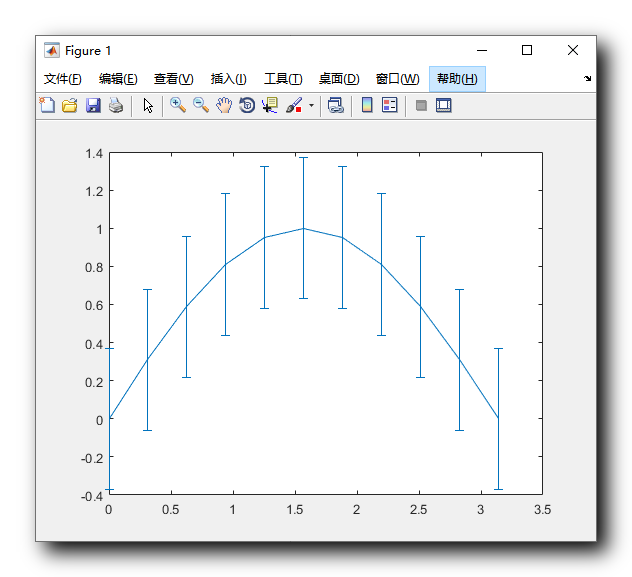展开全文matlab boxplot
• pyecharts中的Bar函数的改动 from pyecharts.charts import Bar #新用法,原来的不能用了from pyecharts import Bar from pyecharts.charts import Grid from pyecharts.charts import Kline from pyecharts.charts ...
pyecharts中的Bar函数的改动
from pyecharts.charts import Bar
#from pyecharts import Bar不能用了
from pyecharts.charts import Grid
from pyecharts.charts import Kline
from pyecharts.charts import WordCloud
from pyecharts.charts import Pie
from pyecharts.charts import Map
Bar包中函数add不能正常使用, 改用
bar.add_xaxis()
bar.add_yaxis()


展开全文python
• matlab利用bar函数画直方图，参考文献是matlab官方提供的help文档。里面提供了bar函数的基本用法，但是没有说明如何在同一张图中，为每个bar设置不同的颜色。 例子代码： mydata=rand(1,5); figure(1) ...
matlab利用bar函数画直方图，参考文献是matlab官方提供的help文档。里面提供了bar函数的基本用法，但是没有说明如何在同一张图中，为每个bar设置不同的颜色。

例子代码：

mydata=rand(1,5);
figure(1)
hold on
for i = 1:length(mydata)
h=bar(i,mydata(i));
if mydata(i) < 0.2
set(h,'FaceColor','k');
elseif mydata(i) < 0.6
set(h,'FaceColor','b');
else
set(h,'FaceColor','r');
end
end
set(gca,'xtick',[1 2 3 4 5]); % 故意为了只是显示5个刻度值,下方的设置才有效
set(gca,'xticklabel',{'直方图1','直方图2','直方图3','直方图4','直方图5'});
hold off

参考文献：

1.《bar》

欢迎关注朋友的公众号“证件照一键换底色”，可处理证件照（换背景、换底色、换正装）展开全文• #将画布分为1行2列 在第一个区域画bar plt.subplot(1,2,1) #添加颜色 plt.bar(x,y,color='blue') #在0位置水平方向添加蓝色的线条 plt.axhline(0,color='blue',linewidth=2) #在第二个区域画barh # barh 将y和x进行...
• ## python bar函数

万次阅读 2017-03-10 21:14:10
bar(left, height, width, color, align, yerr)函数：绘制柱形图。left为x轴的位置序列，一般采用arange函数产生一个序列；height为y轴的数值序列，也就是柱形图的高度，一般就是我们需要展示的数据；width为柱形图...python
• Python的matplotlib模块中的errorbar函数可以绘制误差棒图，本次主要绘制不带折线的误差棒图。 传送门1.基本参数2.代码实现3.结果显示4.更多参数请参考matplotlib官网 1.基本参数 errorbar函数的基本参数主要有： ...python matplotlib numpy
• ## matlab bar函数

千次阅读 2016-05-05 14:26:46
matlab中函数bar绘制直方图中的应用函数bar(x)可以绘制直方图，这对统计或者数据采集非常直观实用。它共有四种形式： bar,bar3,barh 和bar3h，其中barbar3 分别用来绘制二维和三维竖直方图，barh 和b ar3h ...
• 如果Y为矩阵，侧bar函数对每一行元素绘制的条形进行分组。   bar(x,Y) 按x中指定的位置绘制Y中每一元素的条形。   bar(…,width) 设置相邻条形的宽度并控制组内条形的分离，默认值为0.8，如果指定width=1,则组内的...
• ## matlab bar函数用法总结

万次阅读 多人点赞 2020-05-25 22:52:28
bar(y) x=[5 9 11]; subplot(2,2,2) bar3(x,y) subplot(2,2,3) bar(x,y,'grouped') subplot(2,2,4) bar(rand(2,3),0.75,'stack') bar（y,1）直方条会重叠，此时可以将x显示范围设置成0.5-3.5，ticklabel也可以设置 ...matlab
• matlab利用bar函数画直方图，参考文献是matlab官方提供的help文档。里面提供了bar函数的基本用法，但是没有说明如何在同一张图中，为每个bar设置不同的颜色。例子代码：mydata=rand(1,5);figure(1)hold onfor i =...
• matplotlib.pyplot库的知识点之bar函数——绘制条形图 Help on BarContainer in module matplotlib.container object: class BarContainer(Container) | Container for the artists of bar plots (e.g. created by...python 数据可视化
• 函数Bar()–用于绘制柱状图 使用bar绘制柱状图的过程中涉及到中文字体的显示问题 使用动态参数配置方法，指定了中文黑体 import matplotlib as mpl mpl.rcParams['font.sans-serif'] = ['SimHei'] 换成中文字体之后...python
• 导入Bar: from pyecharts.charts import Bar 原来的代码： chart = Bar("中国天气最低气温排行榜") ...错误分析：Bar()函数不能传入参数。而且add()方法已经去除。 修改后代码： chart = Bar() ...
• Matlab中hist、 histc、bar函数的用法及区别：首先给出matlab帮助文件的介绍：HistcSyntax1.bincounts = histc(x,binranges)2.bincounts = histc(x,binranges,dim)3.[bincounts,ind]= histc(___)...
• 参考：　https://blog.csdn.net/jenyzhang/article/details/52047557 　... 需求： 封装一个带分组功能的bar绘制函数 绘制效果： 代码： 1 # -*- coding: utf-8 -*- 2...
• 我们可以利用它方便快捷的进行矩阵运算、绘制函数和数据、图像处理。 前一段时间作者在进行毕业设计时使用MATLAB绘制了论文中的一系列插图（包括曲线图、柱状图等），自我感觉其确实是一个必不可少的实用性工具，...MATLAB plot
• Matlab中hist、 histc、bar函数的用法及区别： 首先给出matlab帮助文件的介绍： Histc Syntax 1.bincounts = histc(x,binranges) 2.bincounts = histc(x,binranges,dim) 3.[bincounts,ind]= histc(___) ......